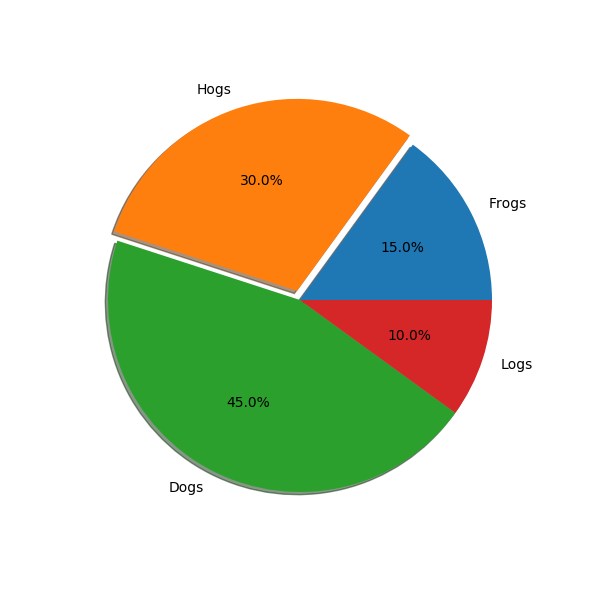Travis-CI:# SVG Filter Pie¶

Demonstrate SVG filtering effects which might be used with mpl. The pie chart drawing code is borrowed from pie_demo.py

Note that the filtering effects are only effective if your svg renderer support it.Out:

```Saving 'svg_filter_pie.svg'
```

```import matplotlib
matplotlib.use("Svg")

import matplotlib.pyplot as plt

# make a square figure and axes
fig1 = plt.figure(1, figsize=(6, 6))
ax = fig1.add_axes([0.1, 0.1, 0.8, 0.8])

labels = 'Frogs', 'Hogs', 'Dogs', 'Logs'
fracs = [15, 30, 45, 10]

explode = (0, 0.05, 0, 0)

# We want to draw the shadow for each pie but we will not use "shadow"
# option as it does'n save the references to the shadow patches.
pies = ax.pie(fracs, explode=explode, labels=labels, autopct='%1.1f%%')

for w in pies:
# set the id with the label.
w.set_gid(w.get_label())

# we don't want to draw the edge of the pie
w.set_ec("none")

for w in pies:
s.set_zorder(w.get_zorder() - 0.1)

# save
from io import BytesIO
f = BytesIO()
plt.savefig(f, format="svg")

import xml.etree.cElementTree as ET

# filter definition for shadow using a gaussian blur
# and lightening effect.
# The lightening filter is copied from http://www.w3.org/TR/SVG/filters.html

# I tested it with Inkscape and Firefox3. "Gaussian blur" is supported
# in both, but the lightening effect only in the Inkscape. Also note
# that, Inkscape's exporting also may not support it.

filter_def = """
<feGaussianBlur result='blur' stdDeviation='2'/>
</filter>

<filter id='MyFilter' filterUnits='objectBoundingBox' x='0' y='0' width='1' height='1'>
<feGaussianBlur in='SourceAlpha' stdDeviation='4%' result='blur'/>
<feOffset in='blur' dx='4%' dy='4%' result='offsetBlur'/>
<feSpecularLighting in='blur' surfaceScale='5' specularConstant='.75'
specularExponent='20' lighting-color='#bbbbbb' result='specOut'>
<fePointLight x='-5000%' y='-10000%' z='20000%'/>
</feSpecularLighting>
<feComposite in='specOut' in2='SourceAlpha' operator='in' result='specOut'/>
<feComposite in='SourceGraphic' in2='specOut' operator='arithmetic'
k1='0' k2='1' k3='1' k4='0'/>
</filter>
</defs>
"""

tree, xmlid = ET.XMLID(f.getvalue())

# insert the filter definition in the svg dom tree.
tree.insert(0, ET.XML(filter_def))

for i, pie_name in enumerate(labels):
pie = xmlid[pie_name]
pie.set("filter", 'url(#MyFilter)')